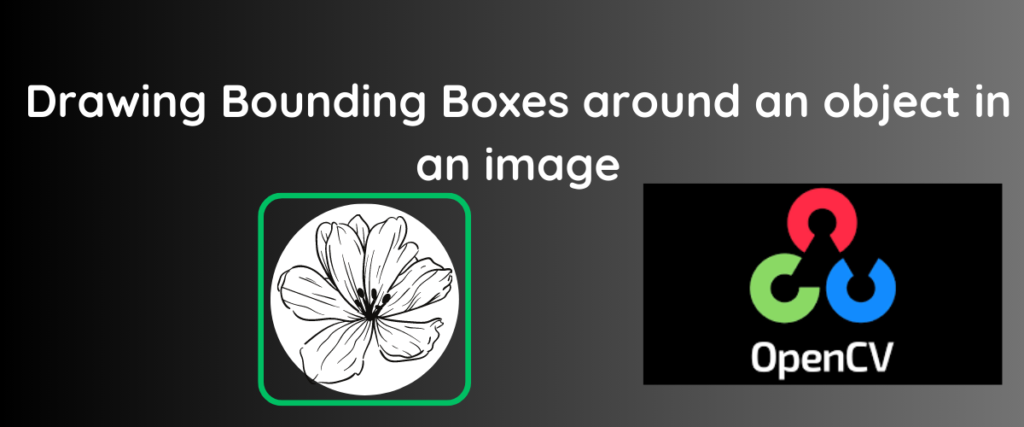# Drawing Bounding Boxes Around Objects in an Image- Easy GuideComputer Vision and Object Detection or Recognition systems use Bounding Boxes or rectangles to identify an object in an image or video frame.

Bounding boxes appear in the boundary of an object distinguishing it from other things, which can be useful for performing object tracking in sequential video frames.

Let me explain with a simple example. Suppose you want to track the movements of a car that has just trespassed in your `No Parking Zone` garden. You reach out to the nearest traffic station to catch the people in the car. What happens there? The officers analyze the traffic in that route from the camera footage and use Computer Vision to draw a boundary for the car that might differentiate it from the other cars. Now every time the car moves to a different place, the officers can track it with the help of the boundary box around it. By this, we can track the car’s recent location and take necessary actions!

In this article, we are going to focus on drawing boundary boxes around multiple objects in a binary image.

## What Is a Binary Image?

A binary image is an image with just two colors usually black and white. In image processing, binary images contain only one bit per pixel, and each pixel is either 0 or 1.

## Prerequisites

Before we move on to drawing boundary boxes, we need to install the OpenCV library first. We can install it with the following command.

```pip install opencv-python
```

## Drawing a Bounding Box for One Object

Let us start off easy. In this example, we are going to take a binary image that has only one object and draw a bounding box to it.

For reference purposes, the image we are using in this example is given below.

The code is given below.

```import cv2
contours, _ = cv2.findContours(bimage, cv2.RETR_EXTERNAL, cv2.CHAIN_APPROX_SIMPLE)
imagewithbbox = cv2.cvtColor(bimage, cv2.COLOR_GRAY2BGR)
for contour in contours:
x, y, w, h = cv2.boundingRect(contour)
cv2.rectangle(imagewithbbox, (x, y), (x + w, y + h), (0, 255, 0), 2)
cv2.imwrite('/content/oneobjectrec.jpg', imagewithbbox)
cv2_imshow(imagewithbbox)
```

We are using the line `from google.colob.patches import...` since in google colaboratory, the `imshow()` method is disabled. If you are not using Google Colab and any other notebook or IDE, you can import the function with import `cv2.imshow()`.

The OpenCV library we installed is imported into the code as cv2. The `cv2_imshow` is imported from the Google colab to avoid any errors.

The binary image we want to draw a boundary for is stored in a variable called image. The image is read with the help of `imread` and is gray-scaled with the help of `cv2.IMREAD_GRAYSCALE`.

The `findContours` is used to find all the continuous points having the same colors or intensities.The `cv2.CHAIN_APPROX_SIMPLE` acts as a flag to ensure that the rectangle is drawn on the required object itself.

In the next line, we create a copy of the image so we can draw a rectangle on the object. We are iterating a for loop on the Contours such that all the objects with contours are recognized, and the boundaries are drawn. The boundingRect function is used to get all the coordinates and the width and height of the rectangle. The code (0,255,0) is a code for specifying the color from the RGB scale. In this case, the bounding rectangle is green in color. The component `2` is used to define the thickness of the box. Here, it is 2 pixels.

We are saving the image with the help of `imwrite` and is displayed with the help of `imshow`.

The output is as follows.

Similarly, we can draw the bounding boxes for two objects too! Let us see a little complex example of drawing a bounding box for three objects.

## Drawing Bounding Boxes for Three Objects

The code is essentially the same. Since we have a for loop iterating over the objects of an image, the number of objects doesn’t really matter. In this code, we are going to draw a red boundary box around the objects.

The image we are going to use is inspired by Harry Potter. The image has HP’s scar, deathly hallows, and sorting hats.

The image is shown below.

Now if we run the code for this image, we get an image with three bounding boxes for the objects. The code is given below.

```import cv2
contours, _ = cv2.findContours(binary_image, cv2.RETR_EXTERNAL, cv2.CHAIN_APPROX_SIMPLE)
image_with_rectangles = binary_image.copy()
image_with_rectangles = cv2.cvtColor(image_with_rectangles, cv2.COLOR_GRAY2BGR)

for contour in contours:
x, y, w, h = cv2.boundingRect(contour)
cv2.rectangle(image_with_rectangles, (x, y), (x + w, y + h), (0,0,255), 6)
cv2.imwrite('/content/hpboundrec.jpg', image_with_rectangles)
cv2_imshow(image_with_rectangles)
```

The image with bounding boxes is shown below.

The small dots around the objects and the bounding boxes could be due to the presence of little contours in the image that could be detected as an object. These are often called noise and can be removed by setting a threshold for contouring.

The code is given below.

```import cv2
contours, _ = cv2.findContours(binary_image, cv2.RETR_EXTERNAL, cv2.CHAIN_APPROX_SIMPLE)
min_contour_area = 100
image_with_rectangles = cv2.cvtColor(binary_image, cv2.COLOR_GRAY2BGR)
for contour in contours:
contour_area = cv2.contourArea(contour)
if contour_area >= min_contour_area:
x, y, w, h = cv2.boundingRect(contour)
cv2.rectangle(image_with_rectangles, (x, y), (x + w, y + h), (0, 0, 255), 6)
cv2.imwrite('/content/hpboundrec.jpg', image_with_rectangles)
cv2_imshow(image_with_rectangles)

```

Observe line 5. We have set a threshold of 100 for contouring. We only draw a bounding rectangle around an object with a contour that is greater than 100. In this way, we can ignore the possible objects with smaller contour areas,